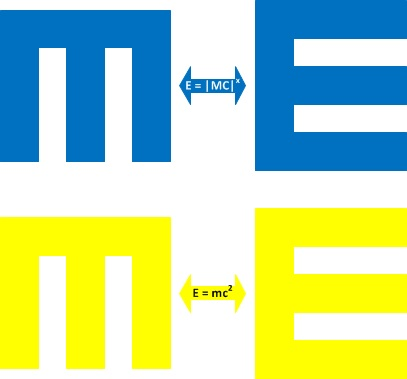Entropy as Memory and Memory as Entropy

Letter transformations sometimes may play the role of analogies and metaphors. One is Mass and Energy and the other is Memory and Entropy. And so we postulate the equivalence between Memory and Entropy where have an equivalence transformation similar to the famous equation E=mc2 which can be written as E=|M|x, where |M| is some not yet specified measure of a Memory container (may be similar to a set-theoretical cardinality). Here we can also write mc as an abbreviation of a memory container. This transformation is illustrated on the following picture:- Dmitry Vostokov - Memoriarch @ MemoryReligion.com -